• C++ 图结构邻接矩阵简单实现
千次阅读
2017-03-31 21:04:25

c++ 图结构邻接矩阵简单实现

#ifndef GRAPHMAT_H
#define GRAPHMAT_H

#include <iostream>
using namespace std;

#define MAX_VERTEX_NUM  20                              //图的最大顶点
#define INF             10000                           //最大极值
typedef enum{ DG, DN, UDG, UDN } GraphKind;                  //图的种类
typedef int TYPE;                                       //数据的类型

class GraphMat
{
public:
GraphMat(GraphKind k);
~GraphMat();
private:
/*
*图的邻接矩阵一般需要6个信息
*1、图的种类。枚举类型:有向图DG，有向网DN，无向图UDG，无向网UDN。
*2、图的顶点个数
*3、顶点的数据值数组GraphMat(GraphKind k) :kind(k), vex_num(0);
*4、图的边（弧）个数
*5、邻接矩阵。对图：用1或0表示两顶点是否相邻；对网：表示两顶点之间的权值。
*6、遍历标志
*/
GraphKind kind;
int vex_num;
TYPE vexs[MAX_VERTEX_NUM];
int arc_num;
int arcs[MAX_VERTEX_NUM][MAX_VERTEX_NUM];
bool is_trav[MAX_VERTEX_NUM];
public:
void CreateGraph();                                 //构造一个图
void BFSTraverse();                                 //广度优先遍历
void DFSTraverse();                                 //深度优先遍历
private:
void BFSFunction(int);                              //广度优先
void DFSFunction(int);                              //深度优先
};

GraphMat::GraphMat(GraphKind k = UDG)
:kind(k), vex_num(0), arc_num(0)
{
//清空矩阵
for (int i = 0; i < MAX_VERTEX_NUM; i++)
{
for (int j = 0; j < MAX_VERTEX_NUM; j++)
arcs[i][j] = INF;                           //设置矩阵中各元素的值为最大值
}
//清空遍历标志
for (int i = 0; i < MAX_VERTEX_NUM; i++){
is_trav[i] = 0;
}
}

GraphMat::~GraphMat()
{
}

void GraphMat::CreateGraph()
{
cout << "输入顶点元素的个数" << endl;
cin >> vex_num;
cout << "输入顶点元素的类型值" << endl;
for (int i = 0; i < vex_num; i++){
cin >> vexs[i];
}

cout << "输入邻接矩阵的大小" << endl;
cin >> arc_num;
cout << "输入邻接矩阵" << endl;
for (int i = 0; i < arc_num; i++){
for (int j = 0; j < arc_num; j++){
cin >> arcs[i][j];
}
}
}

//广度优先遍历
void GraphMat::BFSTraverse()
{
//将标记清0
for (int i = 0; i < vex_num; i++){
is_trav[i] = 0;
}
//对顶点遍历
for (int i = 0; i < vex_num; i++){
//if (!is_trav[i]){
//  BFSFunction(i);
//}
BFSFunction(i);
}
}

void GraphMat::BFSFunction(int i)
{
if (!is_trav[i]){
is_trav[i] = 1;                                     //标记顶点
cout << "->" << vexs[i];
}
for (int j = 0; j < vex_num; j++){
if (arcs[i][j] != 0 && !is_trav[j]){
cout << "->" << vexs[j];
is_trav[j] = 1;
}
}
}

void GraphMat::DFSTraverse()
{
//将标记清0
for (int i = 0; i < vex_num; i++){
is_trav[i] = 0;
}
//对顶点遍历
for (int i = 0; i < vex_num; i++){
if (!is_trav[i]){
DFSFunction(i);
}
}
}

void GraphMat::DFSFunction(int i)
{
is_trav[i] = 1;
cout << "->" << vexs[i];
for (int j = 0; j < vex_num; j++){
if (arcs[i][j] != 0 && !is_trav[j]){
DFSFunction(j);
}
}
}
#endif

测试用例：

// Graph.cpp : 定义控制台应用程序的入口点。
//

#include "stdafx.h"
#include <iostream>
#include "GraphMat.h"
using namespace std;

int _tmain(int argc, _TCHAR* argv[])
{
GraphMat graph(UDG);
graph.CreateGraph();
cout << "广度遍历效果 ： " << endl;
graph.BFSTraverse();
cout << endl;
cout << "深度遍历效果 ： " << endl;
graph.DFSTraverse();
cout << endl;
return 0;
}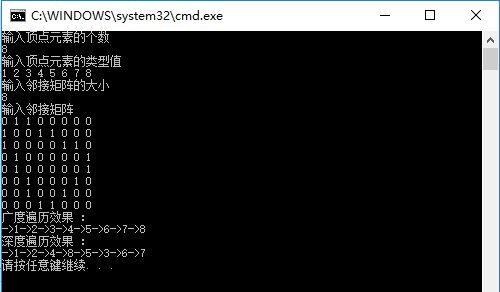更多相关内容
• 领会的两种主要存储结构基本运算算法和两种遍历算法设计内容：编写一个程序，设计带权邻接矩阵与邻接表的创建和输出运算，并在此基础上设计一个主程序完成如下功能：（1）建立如所示的有向G的邻接矩阵...
• 主讲人 李 刚 邻接矩阵表示法 C 目 录 ONTENTS 01 邻接矩阵的定义 邻接矩阵的定义 1 邻接矩阵是表示顶点之间相邻关系的矩阵设G是具有n个顶点的无论是有向还是无向则G的邻接矩阵是具有如下性质的n阶方阵 ...
• 主要为大家详细介绍了C语言实现邻接矩阵存储操作，具有一定的参考价值，感兴趣的小伙伴们可以参考一下
• 博文测试代码，博文链接：https://blog.csdn.net/qq_44075108/article/details/116085013
• 仅限学习使用 个人资料整理 数学与计算机学院 课程设计说明书 数据结构与算法课程设计名 称: 课程 : 6014389 码程 代 课 目: 无向邻接矩阵存储结构题 4班 级软件年级专业班: 2018// 名: 吴超学 生 姓 号: ...
• Prim算法计算最小生成树（无向&邻接矩阵）——C语言实现。
• 3. 构造邻接矩阵和顶点集。 4. 输出的各顶点和邻接矩阵 5. 插入一条边 6. 删除一条边 7. 求出各顶点的度 8. 判断该是否是连通，若是，返回1；否则返回0. 9. 使用深度遍历算法，输出遍历序列
• 自行实现邻接矩阵和邻接表存储结构 邻接矩阵类和邻接表类的实现及测试函数 功能全 代码易理解 可直接运行
• 数据结构图-邻接矩阵的介绍及实现 本文简单介绍了的几种类型以及的数据结构，并利用C++构建工程实现了有向和无向 的基本定义 是一种多对多的数据结构，由顶点和边构成 带/无权：如果的边的长度不...

# 数据结构图-邻接矩阵的介绍及实现

本文简单介绍了图的几种类型以及图的数据结构，并利用C++构建工程实现了有向图和无向图

## 图的基本定义

图是一种多对多的数据结构，由顶点和边构成

1. 带/无权图：如果图的边的长度不完全相同，则图为带权图。有/无向图：如果给图的每条边规定一个方向，那么得到的图称为有向图。在有向图中，与一个节点相关联的边有出边和入边之分，而与一个有向边关联的两个点也有始点和终点之分。相反，边没有方向的图称为无向图。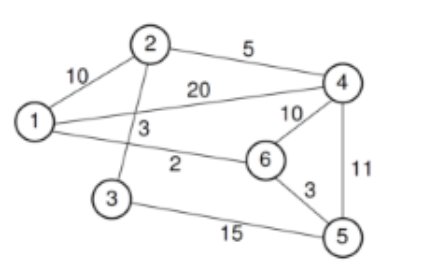2. 有/无向图：如果给图的每条边规定一个方向，那么得到的图称 为有向图。在有向图中，与一个节点相关联的边有出边和入边之分，而与一个有向边关联的两个点也有始点和终点之分。相反，边没有方向的图称为无向图。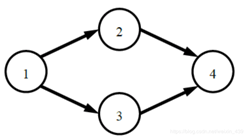## 邻接矩阵

#### 1.简单介绍

用一个一维数组存放图中所有顶点数据；用一个二维数组存放顶点间关系（边或弧）的数据，这个二维数组称为邻接矩阵。用邻接矩阵表示图，很容易确定图中任意两个顶点是否有边相连。邻接矩阵分为有向图邻接矩阵和无向图邻接矩阵。对无向图（无向简单图）而言，邻接矩阵一定是对称的，而且对角线一定为零，有向图则不一定如此。

#### 2.无向图的邻接矩阵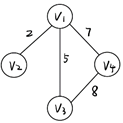图的顶点数为n，边数为m

• 无向图的邻接矩阵

• 利用一个一维数组V[n-1]存储顶点下标
• 利用一个二维数组A[n][n],其中A[i][j]中存放的是顶点i到顶点j的距离
• 若i=j则距离为0（邻接矩阵的对焦新为0）
• 若i与j之间不存在边，则设为∞（后文代码中设为0）
• 否则设为边长

该图可以得到如下的临接矩阵，v1->v2为2，因此A=2.A=2,V2与V3之间没有边，A=A=∞其余顶点同理。有向图的同理。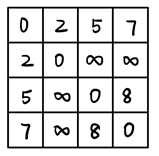## 图的实现

将无向图与有向图放在一个工程中实现，利用一个字符型变量UnDirected来区分有向图和无向图。当Directed为D时构建有向图，否则构建无向图。
（天我真的好喜欢免费分享的我自己hhh打扰了）

##### 实现工程
###### Graph.h
#ifndef GRAPH
#define GRAPH
template<typename VertexType>//类模板
class Graph
{
private:
void operator=(const Graph&){}
Graph(const Graph&){}
public:
Graph(){}
virtual ~Graph(){}								//默认构造函数
virtual void Init(int n)=0;						//析构函数
virtual int n()=0;								//返回顶点数
virtual int e()=0;								//返回边数
virtual int first(int v)=0;						//返回顶点v的邻居
virtual int next(int v,int w)=0;				//返回在w之后的邻居
virtual void SetType(bool flag)=0;				//设置图的类型，有向图或无向图
virtual bool GetType()=0;						//获取图的类型，有向或无向图
virtual int LocateVex(VertexType u)=0;			//找到顶点在图中的位置
virtual VertexType GetVex(int v)=0;				//返回某个顶点的值
virtual void PutVex(int v,VertexType value)=0;	// 给某个顶点赋值
virtual void SetEdge(int v1,int v2,int wght)=0;	//设置边长
virtual void DelEdge(int v1,int v2)=0;			//删除边
virtual bool IsEdge(int i,int j)=0;				//两个顶点之间是否存在边
virtual int weight(int v1,int v2)=0;			//边的权重
virtual int GetMark(int v)=0;					//获取该顶点是否被访问过的标记
virtual void SetMark(int v,int val)=0;			//设置是否被访问过的标记
};
#endif

###### Graphm.h
#include<iostream>
#include"graph.h"
#define MAX_VERTEX_NUM 40
#define UNVISITED 0
#define VISITED 1
using namespace std;

template<typename VertexType>
class Graphm:public Graph<VertexType>
{
private:
int NumVertex,NumEdge;				//顶点数和边数
bool UnDirected;					//true表示无向图，false表示有向图
VertexType vexs[MAX_VERTEX_NUM];	//存储顶点信息
int **matrix;						//指向邻接矩阵matrix
int *mark;							//标记矩阵，指向mark数组
public:
Graphm(int NumVert)			//构造函数
{
Init(NumVert);
}
~Graphm()
{
delete[]mark;
for(int i=0;i<NumVertex;i++)
{
delete[]matrix[i];
delete[]matrix;
}
}
void Init(int n)//初始化图
{
int i;
NumVertex=n;
NumEdge=0;
mark=new int[n];//初始化mark数组
for(i=0;i<NumVertex;i++)
{
mark[i]=UNVISITED;
}
matrix=(int**)new int*[NumVertex];
for(i=0;i<NumVertex;i++)
{
matrix[i]=new int[NumVertex];
}
for(i=0;i<NumVertex;i++)
{
for(int j=0;j<NumVertex;j++)
matrix[i][j]=0;
}
}
int n()
{
return NumVertex;//返回顶点数
}
int e()//返回边数
{
return NumEdge;
}
int first(int v)//获取顶点v 的第一条边
{
for(int i=0;i<NumVertex;i++)
{
if(matrix[v][i]!=0)return i;

}
return NumVertex;//若无，返回顶点数
}
int next(int v,int w)//获取顶点v在顶点w后的第一条边
{
for(int i=w+1;i<NumVertex;i++)
{
if(matrix[v][i]!=0)//若顶点间存在边
return i;

}
return NumVertex;//若无返回顶点数
}
void SetType(bool flag)//设置图的类型，有向图或无向图
{
UnDirected=flag;
}
bool GetType()//获取图的类型
{
return UnDirected;
}
int LocateVex(VertexType u)//返回顶点在图中的位置
{
for(int i=0;i<NumVertex;i++)
{
if(u==vexs[i])return i;
}
return -1;
}
VertexType GetVex(int v)//返回某个顶点的值
{
return vexs[v];
}
void PutVex(int v,VertexType value)//给顶v点值
{
vexs[v]=value;
}
void SetEdge(int v1,int v2,int wt)//设置v1到v2的边，权为wt
{
if(wt<0)return;
if(matrix[v1][v2]==0)NumEdge++;
matrix[v1][v2]=wt;
if(UnDirected)//若为无向图，在v2到v1也设置边
{
matrix[v2][v1]=wt;
}
}
void DelEdge(int v1,int v2)//删除边
{
if(matrix[v1][v2]!=0)
{
NumEdge--;
matrix[v1][v2]=0;
if(UnDirected)
{
matrix[v2][v1]=0;//若为无向图，则同时删除从v2->v1的边
}
}
}
bool IsEdge(int i,int j)//判断是否存在边
{
return matrix[i][j]!=0;
}
int weight(int v1,int v2)//获取边的权重
{
return matrix[v1][v2];
}
int GetMark(int v)//获取标记
{
return mark[v];
}
void SetMark(int v,int val)//设置标记
{
mark[v]=val;
}
};

###### main.cpp

建图思路：输入顶点数，初始化相应大小的二维数组。由给出的每条边的信息(起点，终点，权值)，将对应起点终点和终点起点下标的两个数组位置赋值为权值，依次处理每一条边的信息。
构建方法：输入顶点数以及边数，利用顶点数构建一个循环输入顶点信息构建顶点，再利用边数构建一个循环设置两个顶点之间的边的权重。

#include<iostream>
#include<cstring>
#include "graphm.h"
#include <windows.h>
using namespace std;
void Gprint(Graphm<string>* G)
{
int i, j;
cout << "顶点数:" <<G->n() << "\n";
cout << "边  数:" <<G->e() << "\n";
cout << "图类型:"<<(G->GetType()?"无向图":"有向图")<<endl;
cout << "输出顶点信息：\n";
for(i=0; i<G->n(); i++)
{
cout<<G->GetVex(i)<<"  ";
}
cout<<endl;
cout<<"输出边信息:\n";
if(G->GetType()) //若为无向图
{
for (i=0;i<G->n();i++)
{
for(j=i;j<G->n();j++)
{
if(G->weight(i,j)!=0) //若边存在
{
cout<<G->GetVex(i)<<"<-->"<<G->GetVex(j)<<":"<<G->weight(i,j)<<endl;
}
//输出两个顶点以及之间的边
}
}
}
else //有向图
{
for (i=0;i<G->n();i++)
{
for(j=0;j<G->n();j++)
{
if(G->weight(i,j)!=0)
{
cout<<G->GetVex(i)<<"-->"<<G->GetVex(j)<<":"<<G->weight(i,j)<<endl;
}
}
}
}
cout <<"邻接矩阵为:\n";
for (i=0;i<G->n();i++)
{
for(j=0;j<G->n();j++)
cout <<G->weight(i, j)<<" ";

cout << "\n";
}
}
int main()
{
int num,num_edge;
cout<<"请输入顶点个数:";
cin>>num;
Graphm<string>*G=new Graphm<string>(num);
string ver;
cout<<"请输入顶点信息，并用空格隔开:";
for(int i=0;i<num;i++)
{
cin>>ver;
G->PutVex(i,ver);
}
cout<<"是否为有向图，D/U:";
char ch;
cin>>ch;
if (ch=='U') G->SetType(true);//无向图
else if (ch=='D') G->SetType(false);//有向图
cout<<"请输入边数:";
cin>>num_edge;
cout<<"请输入边:"<<endl;
string s1,s2;
int v1,v2,dist;
for(int i=0;i<num_edge;i++)
{
cin>>s1;
v1=G->LocateVex(s1);
cin>>s2;
v2=G->LocateVex(s2);
cin>>dist;
G->SetEdge(v1,v2,dist);
}
Gprint(G);
return 0;
}


##### 运行结果
###### 输入部分

输入顶点个数为5，信息分别为ABCDE，设置UNDirected为D，即为建立一个有向带，再输入边的条数，最后存在边的顶点以及边的权值。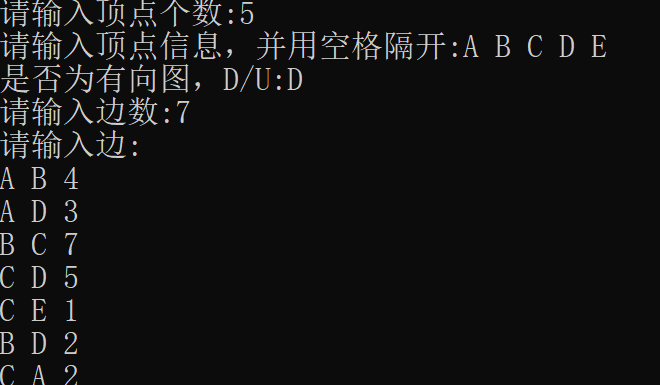###### 输出部分

与输入部分进行对照，结果正确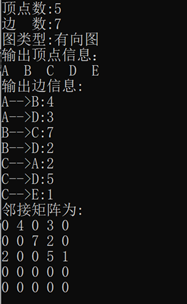展开全文c++ 算法
• 邻接矩阵的方式 Python代码示例 # !/usr/bin/env python # -*-encoding: utf-8-*- # author:LiYanwei # version:0.1 # 邻接矩阵 ''' a---b\ | | \ | | c | | / e---d/ 对于无向顶点之间存在边,则为1,反之则为0 a...
• 数据结构图邻接矩阵,邻接表存储表示，的深度优先搜索遍历，广度优先搜索遍历 数据结构图邻接矩阵,邻接表存储表示，的深度优先搜索遍历，广度优先搜索遍历.rar
• 主要介绍了Java编程实现邻接矩阵表示稠密代码示例，具有一定参考价值，需要的朋友可以了解下。
• 的邻接表 邻接矩阵表示的迪杰斯特拉算法 普里姆算法 克鲁斯卡尔算法 用c++实现 codeblocks编译通过
• 的存储结构邻接矩阵表示法存储表示采用邻接矩阵表示法创建无向网用代码实现无向网 若有n个顶点，就是n*n的方阵，与边数无关 对称矩阵：从矩阵的左上角到右上角的主对角线为轴，右上角的元与左下角对应的元全都是...

## 邻接矩阵表示法

若有n个顶点，就是n*n的方阵，与边数无关

对称矩阵：从矩阵的左上角到右上角的主对角线为轴，右上角的元与左下角对应的元全都是相等的

有向图、无向图的邻接矩阵表示法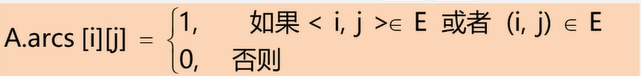如：
无向图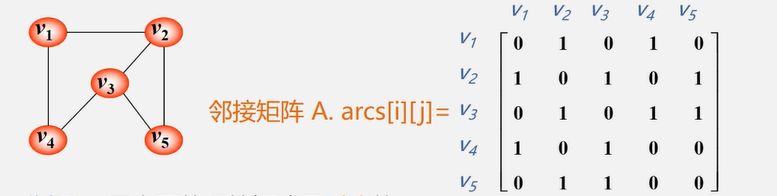总结：

1. 无向图的邻接矩阵是对称的
2. 顶点i的度=第i行（列）中1的个数；
3. 特别的：完全图的邻接矩阵中，对角元素为0，其余为1；

有向图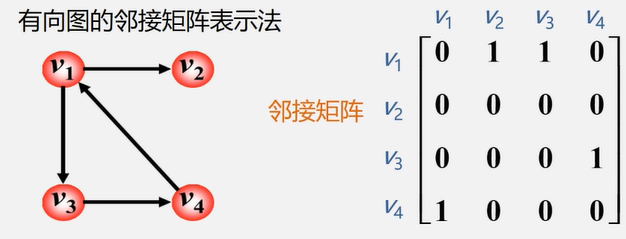总结：

1. 第i行含义：以结点vi为尾的弧（即出度边）
2. 第i列含义：以结点vi为头的弧（即入度边）
3. 有向图的邻接矩阵可能是不对称的
4. 顶点的出度=第i行元素之和
5. 顶点的入度=第i列元素之和
6. 顶点的度=第i行元素之和+第i列元素之和

网的邻接矩阵表示法

定义为：如：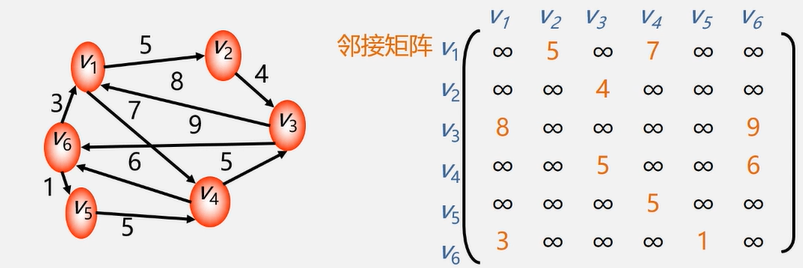## 存储表示

顶点表用一维数组存储、邻接矩阵用二维数组存储

两个数组分别存储顶点表邻接矩阵（也叫边表）

#define MaxInt 32767 //表示极大值，即无穷
#define MVNum 100	//最大顶点数
typedef char VerTexType;	//设顶点的数据类型为字符型
typedef int ArcType;	//假设边的权值类型为整形

typedef struct{
VerTexType vexs[MVNum];	//顶点表
ArcType arcs[MVNum][MVNum];	//邻接矩阵,可以看作边表
int vexnum,arcnum;	//图的当前点数和边数



## 采用邻接矩阵表示法创建无向网

已知一个图的点和边，使用邻接矩阵表示法来创建此图的方法比较简单，以无向网为例

【算法思想】

1. 输入总顶点数和边数
2. 依次输入点的信息存入顶点表中。
3. 初始化邻接矩阵，使得每个权值初始化为极大值
4. 构造邻接矩阵

依次输入每条边依附的顶点和其权值

确定两个顶点在图中的位置之后，使相应边赋予相应的权值

同时使其对称边赋予相同的权值

补充一下顶点表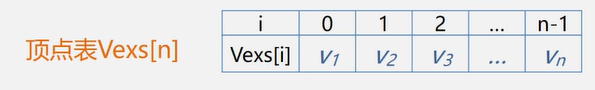【算法描述】

bool CreateUDN(AMGraph &G)
{
char v1,v2;
int w,i,j,k;
cin>>G.vexnum>>G.arcnum; //输入总顶点数，总边数
for(i=0;i<G.vexnum;i++)//依次输入点的信息
cin>>G.vexs[i];
for(i=0;i<G.vexnum;i++)
for(j=0;j<G.vexnum;j++)
G.arcs[i][j]=MAXInt;//初始化邻接矩阵，边的权值均置为极大值MAXInt
for(k=0;k<G.arcnum;k++){//构造邻接矩阵
cin>>v1>>v2>>w;//输入一条边所依附的顶点及边的权值
i=LocateVex(G,v1);
j=LocateVex(G,v2);//确定v1和v2在G中的位置，即顶点数组的下标
G.arcs[i][j]=w; //边<v1,v2>权值置为w
G.arcs[j][i]=G.arcs[i][j];  //边<v1,v2>的对称边<v2,v1>的权值为w
}//for
return true;
}//CreateUDN



注：从代码中可以得到，n个顶点和e条边的无向网图的创建，时间复杂度度为O(n+ n ^2 + e ), 其中对邻接矩阵G.arc的初始化耗费了O(n ^ 2)的时间。

【查找顶点的算法】

int LocateVex(AMGraph G,VertexType u){
//在图G中查找顶点u，存在则返回顶点表中的下标；否则返回-1
int i;
for(i=0;i<G.vexnum;i++)
if(u==G.vexs[i])
return i;
return -1;
}


建立无向网后，想要建立无向图，有向网和有向图时只需要稍稍改动即可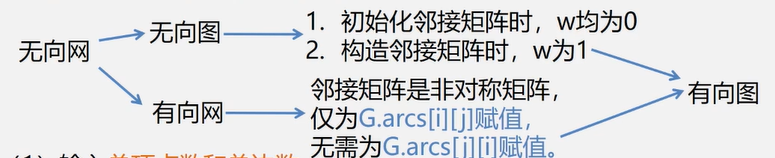## 用代码实现无向网

#include<iostream>
using namespace std;
#define MaxInt 32767 //表示极大值，即无穷
#define MVNum 100 //最大顶点数
typedef char VerTexType; //设顶点的数据类型为字符型
typedef int ArcType; //假设边的权值类型为整形

typedef struct{
VerTexType vexs[MVNum]; //顶点表
ArcType arcs[MVNum][MVNum]; //邻接矩阵
int vexnum,arcnum; //图的当前点数和边数

//在图中查找顶点
int LocateVex(AMGraph G,VerTexType u){
//在图G中查找顶点u，存在则返回顶点表中的下标；否则返回-1
int i;
for(i=0;i<G.vexnum;i++)
if(u==G.vexs[i])
return i;
return -1;
}
//采用邻接矩阵表示法，创建无向网G
bool CreateUDN(AMGraph &G)
{
char v1,v2;
int w,i,j,k;
cout<<"分别输入总顶点数、总边数：";
cin>>G.vexnum>>G.arcnum; //输入总顶点数，总边数
cout<<"输入点的信息：";
for(i=0;i<G.vexnum;i++)//依次输入点的信息
cin>>G.vexs[i];
for(i=0;i<G.vexnum;i++)
for(j=0;j<G.vexnum;j++)
G.arcs[i][j]=MaxInt;//初始化邻接矩阵，边的权值均置为极大值MAXInt
cout<<"请输入一条边所依附的两个顶点、权值：";
for(k=0;k<G.arcnum;k++){//构造邻接矩阵
cin>>v1>>v2>>w;//输入一条边所依附的顶点及边的权值
int i=LocateVex(G,v1);
int j=LocateVex(G,v2);//确定v1和v2在G中的位置，即顶点数组的下标
G.arcs[i][j]=w; //边<v1,v2>权值置为w
G.arcs[j][i]=G.arcs[i][j];
//边<v1,v2>的对称边<v2,v1>的权值为w
}//for
return true;
}//CreateUDN

int main()
{
AMGraph G;
CreateUDN(G);
cout<<"无向网为：";
for(int i=0;i<G.vexnum;i++)
{

for(int j=0;j<G.vexnum;j++){
cout<<G.arcs[i][j]<<" ";
}
cout<<endl;
}//for
return 0;
}



实现结果为：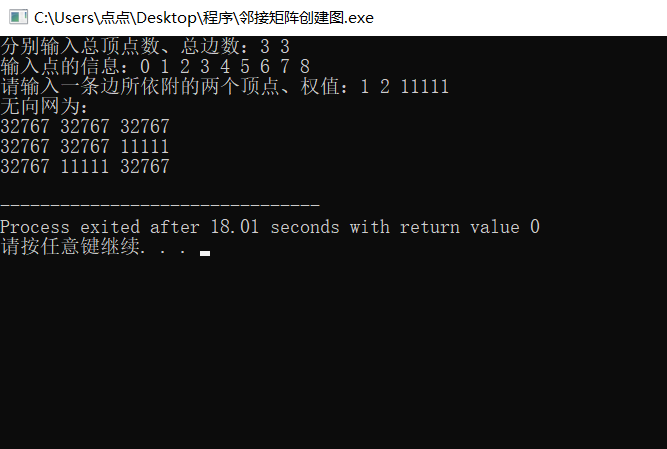优点

1. 直观、简洁，便于判断两个顶点是否有边。
2. 便于计算各顶点的度
3. 方便找任意顶点的邻接点

缺点

1. 不便于增加和删除顶点
2. 浪费空间——存稀疏图（点多边少）有大量无效元素，空间复杂度为O(n^2),存稠密图还是很合算。
3. 浪费时间——统计稀疏图中一共有多少条边，时间复杂度为O(n^2).
展开全文• 利用networkx，numpy，matplotlib，将邻接矩阵输出为图形。 1，自身确定一个邻接矩阵，然后通过循环的方式添加变，然后输出图像 import networkx as nx import matplotlib.pyplot as plt import numpy as np G = nx...
• 利用python载入邻接矩阵绘制网络，基于python语言的特点，对邻接矩阵加以处理后再进行应用，即将邻接矩阵去除第一列（节点序号列），复杂网络的基本拓扑结构可以用图论的方法表示成G =(V，E)，V中元素称为节点或...
•代码
• 数据结构邻接矩阵存储

## 1、 图的存储方法：邻接矩阵存储(无向图)

测试数据：
4 5
A B C D
0 1
0 2
0 3
1 2
1 3

邻接矩阵：

顶点ABCD
A0111
B1011
C1100
D1100

#include<iostream>
#include<malloc.h>
using namespace std;     //vertex:顶点    arc:弧    adjacent:邻近的
#define MaxVertexNum 100  //最大顶点数
typedef char VertexType;  //顶点的数据类型
typedef int EdgeType;   //边的数据类型
typedef struct{
VertexType Vex[MaxVertexNum];  //定点表
EdgeType  Edge[MaxVertexNum][MaxVertexNum];  //邻接矩阵，边表
int vernum,arcnum; //图的当前顶点数和弧数
}MGraph; //适合存储稠密图

//邻接矩阵法创建一个图
void CreateGraph(MGraph *G)
{
int i,j,k,w;
printf("请输入图的顶点数和边数：");
cin>>G->vernum>>G->arcnum;
printf("请输入图的各个顶点的信息（A,B…）：");
for(i=0;i<G->vernum;i++)
cin>>G->Vex[i];//"%c"中%c后面要有空格
for(i=0;i<G->vernum;i++)
{
for(j=0;j<G->vernum;j++)
G->Edge[i][j]=0;
}
printf("请输入各条边的信息（例：1 2表示在A顶点和B顶点之间有一条边）:\n");
for(k=0;k<G->arcnum;k++)
{//此为创建有向图的邻接矩阵
int Iindex,Jindex;
cin>>Iindex>>Jindex;
G->Edge[Iindex][Jindex]=1;
//如果加上G->Edge[j][i]=1;则建立的是无向图的邻接矩阵
G->Edge[Jindex][Iindex]=1;
}
}

//打印邻接矩阵
void DisplayMGraph(MGraph G)
{
int i,j;
printf("	");
for(i=0;i<G.vernum;i++)
printf("%c ",G.Vex[i]);
for(i=0;i<G.vernum;i++)
{
printf("\n%c\t",G.Vex[i]);
for(j=0;j<G.vernum;j++)
printf("%d ",G.Edge[i][j]);
}
}

//求图G中顶点x的第一个邻接点
int FirstNeighbor(MGraph G,char x)
{
int i,j;
for(i=0;i<G.vernum;i++)//找到x在顶点表中的位置
{
if(x==G.Vex[i])
break;
}
for(j=0;j<G.vernum;j++)//在邻接矩阵中找到x所在行的值为1的元素。
{
if(G.Edge[i][j]==1)
break;
}
if(j<G.vernum)
return j;
else
return -1;
}

//求图G中顶点y是顶点x的一个邻接点，返回除y外顶点x的下一个邻接点的顶点号
//若y是x的最后一个邻接点，则返回-1
int NextNeighbor(MGraph G, char x,char y)
{
int i,j,k;
for(i=0;i<G.vernum;i++)
{
if(x==G.Vex[i])
break;
}
for(j=0;j<G.vernum;j++)//在邻接矩阵中找到x所在行的值为1的元素。
{
if(G.Vex[j]==y&&G.Edge[i][j]==1)
break;
}
for(k=j+1;k<G.vernum;k++)
if(G.Edge[i][k]==1)
break;
if(k<=G.vernum)
return k;
else
return -1;
}

int main()
{
MGraph G;
CreateGraph(&G);
printf("图的邻接矩阵如下：\n");
DisplayMGraph(G);
int index1=FirstNeighbor(G,'A');
if(index1!=-1)
printf("\n顶点A的第一个邻接点是%c\n",G.Vex[index1]);
else
printf("顶点A没有邻接点！\n");
int index2=NextNeighbor(G,'A','C');
if(index2!=-1)
printf("\n顶点A的第一个邻接点是%c\n",G.Vex[index2]);
else
printf("顶点C是顶点A的最后一个邻接点！\n");
return 0;
}


## 运行截图展开全文• ## 数据结构图的邻接矩阵

万次阅读 多人点赞 2018-05-07 21:19:59
邻接矩阵的存储方式是用两个数组来实现的，一个一维数组存储顶点信息，一个二维数组存储线（无向）或弧（有向）的信息。设G有n个顶点，则邻接矩阵是一个n × n的方阵，定义为：无向邻接矩阵，两个顶点...
• 3. 构造邻接矩阵和顶点集。 4. 输出的各顶点和邻接矩阵 5. 插入一条边 6. 删除一条边 7. 求出各顶点的度 8. 判断该是否是连通，若是，返回1；否则返回0. 9. 使用深度遍历算法，输出遍历序列
• Python语言，用邻接矩阵实现 通过二维数组建立无向 通过二维数组建立有向 通过边建立有向 为方便查看，通过NetworkX显示
• 前面我们学习图的有些定义和术语，对图这个数据结构有了新的见解和认知，让我们理解图结构的知识，今天我们学习图的存储结构，图的存储结构比较多，我们今天主要是学习邻接矩阵和邻接表，对于邻接矩阵是使用数组的...后端 c语言 算法
•python
• 邻接矩阵 邻接矩阵（Adjacency Matrix）存储⽅式是⽤两个数组来表示。...//邻接矩阵存储结构 typedef struct Graph{ //顶点表 vector<string> Vexs; //边表，表示顶点与顶点之间的...
• c语言写的有向图邻接矩阵的实现，通过使用邻接矩阵实现的存储结构存储。
• 1.采用邻接矩阵实现无向的存储，并输入输出邻接矩阵。求每个顶点的度，并实现的广度优先遍历和深度优先遍历...